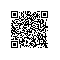# NeHe OpenGL教程 第三十八课：资源文件

NeHe OpenGL第三十八课：资源文件#include <windows.h>
#include <gl\gl.h>
#include <gl\glu.h>
#include <gl\glaux.h>
#include "NeHeGL.h"
#include "resource.h"          // 资源文件的头文件

#pragma comment( lib, "opengl32.lib" )
#pragma comment( lib, "glu32.lib" )
#pragma comment( lib, "glaux.lib" )

GL_Window* g_window;
Keys*  g_keys;

tex将跟踪每个物体所用纹理，x是物体的x坐标，y是y坐标，z,z坐标，yi是一个随机数用来控制物体下落速度，
spinz用来控制沿z轴的旋转，spinzi是另一个随机树，记录旋转速度。flap用来控制物体的翅膀（一会在解释这个）随机数fi控制翅膀拍打的速度。

// 定义三个保存纹理变量的ID
GLuint texture;           // 保存三个纹理

struct object           // 定义一个物体
{
int   tex;          // 纹理值
float x;           // 位置
float y;
float z;
float yi;           // 速度
float spinz;          // 沿Z轴旋转的角度和速度
float spinzi;
float flap;          // 是否翻转三角形
float fi;
};

object obj;           // 创建50个物体

void SetObject(int loop)         // 循环设置50个物体
{
obj[loop].tex=rand()%3;        // 纹理
obj[loop].x=rand()%34-17.0f;       // 位置
obj[loop].y=18.0f;
obj[loop].z=-((rand()%30000/1000.0f)+10.0f);
obj[loop].spinzi=(rand()%10000)/5000.0f-1.0f;      // 旋转
obj[loop].flap=0.0f;
obj[loop].fi=0.05f+(rand()%100)/1000.0f;
obj[loop].yi=0.001f+(rand()%1000)/10000.0f;
}

{
HBITMAP hBMP;          // 位图句柄
BITMAP BMP;          // 位图结构

// 纹理句柄
byte Texture[]={ IDB_BUTTERFLY1, IDB_BUTTERFLY2, IDB_BUTTERFLY3 };

glGenTextures(sizeof(Texture), &texture);       // 创建三个纹理
for (int loop=0; loop<sizeof(Texture); loop++)      // 循环载入所有的位图
{

glPixelStorei告诉OpenGL这些数据是以word alignments存储的，也就是每像素4字节。

。

if (hBMP)         // 位图是否存在
{         // 存在
GetObject(hBMP,sizeof(BMP), &BMP);     // 获得位图
glPixelStorei(GL_UNPACK_ALIGNMENT,4);    // 以四字节方式对其内存
glBindTexture(GL_TEXTURE_2D, texture[loop]);    // 绑定位图
glTexParameteri(GL_TEXTURE_2D,GL_TEXTURE_MAG_FILTER,GL_LINEAR);  // 设置纹理过滤器
glTexParameteri(GL_TEXTURE_2D,GL_TEXTURE_MIN_FILTER,GL_LINEAR_MIPMAP_LINEAR);
// 创建纹理
gluBuild2DMipmaps(GL_TEXTURE_2D, 3, BMP.bmWidth, BMP.bmHeight, GL_BGR_EXT, GL_UNSIGNED_BYTE, BMP.bmBits);
DeleteObject(hBMP);      // 删除位图对象
}
}
}

BOOL Initialize (GL_Window* window, Keys* keys)       // 初始化
{
g_window = window;
g_keys  = keys;

glClearColor (0.0f, 0.0f, 0.0f, 0.5f);       // 设置背景
glClearDepth (1.0f);
glDepthFunc (GL_LEQUAL);
glDisable(GL_DEPTH_TEST);         // 启用深度测试
glHint (GL_PERSPECTIVE_CORRECTION_HINT, GL_NICEST);
glEnable(GL_TEXTURE_2D);         // 启用2D纹理
glBlendFunc(GL_ONE,GL_SRC_ALPHA);        // 使用混合
glEnable(GL_BLEND);

for (int loop=0; loop<50; loop++)
{
SetObject(loop);
}

return TRUE;          // 成功返回
}

void Deinitialize (void)
{
}

void Update (DWORD milliseconds)         // 更新,执行动画
{
if (g_keys->keyDown [VK_ESCAPE] == TRUE)       // 按ESC退出
{
TerminateApplication (g_window);
}

if (g_keys->keyDown [VK_F1] == TRUE)        // 按F1切换显示模式
{
ToggleFullscreen (g_window);
}
}

，大概是指某一种参数化的方法，简单地说使得扭曲最小。）

void Draw (void)          // 绘制场景
{
glClear (GL_COLOR_BUFFER_BIT | GL_DEPTH_BUFFER_BIT);

for (int loop=0; loop<50; loop++)
{

glBindTexture(GL_TEXTURE_2D, texture[obj[loop].tex]);    // 绑定纹理
glTranslatef(obj[loop].x,obj[loop].y,obj[loop].z);    // 绘制物体
glRotatef(45.0f,1.0f,0.0f,0.0f);
glRotatef((obj[loop].spinz),0.0f,0.0f,1.0f);glBegin(GL_TRIANGLES);

glTexCoord2f(1.0f,1.0f); glVertex3f( 1.0f, 1.0f, 0.0f);
glTexCoord2f(0.0f,1.0f); glVertex3f(-1.0f, 1.0f, obj[loop].flap);
glTexCoord2f(0.0f,0.0f); glVertex3f(-1.0f,-1.0f, 0.0f);glTexCoord2f(1.0f,1.0f); glVertex3f( 1.0f, 1.0f, 0.0f);
glTexCoord2f(0.0f,0.0f); glVertex3f(-1.0f,-1.0f, 0.0f);
glTexCoord2f(1.0f,0.0f); glVertex3f( 1.0f,-1.0f, obj[loop].flap);

glEnd();

//移动，选择图像
obj[loop].y-=obj[loop].yi;
obj[loop].spinz+=obj[loop].spinzi;
obj[loop].flap+=obj[loop].fi;

if (obj[loop].y<-18.0f)        //判断是否超出了屏幕，如果是重置它
{
SetObject(loop);
}

if ((obj[loop].flap>1.0f) || (obj[loop].flap<-1.0f))
{
obj[loop].fi=-obj[loop].fi;
}
}

Sleep(15);

glFlush ();
}

http://nehe.gamedev.net/data/lessons/lesson.asp?lesson=38

本文转自wenglabs博客园博客，原文链接：http://www.cnblogs.com/arxive/p/6239541.html，如需转载请自行联系原作者使用钉钉扫一扫加入圈子
+ 订阅